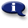# .

## Syllabus Information

Spring 2022
Sep 30,2023Use this page to maintain syllabus information, learning objectives, required materials, and technical requirements for the course.

Syllabus Information
MTH 065D - Elementary Algebra: Part D
Associated Term: Spring 2022
Learning Objectives: Upon successful completion of this course, the student should be able to: 1. Maintain, use, and expand skills and concepts learned in previous mathematics courses. Perform addition, subtraction, multiplication, and division of rational numbers. Use and apply the concepts and language of algebraic expressions. Solve linear equations and inequalities. Interpret information represented numerically and graphically, and recognize the linear relationships represented verbally, numerically, graphically, and algebraically. 2. Use information given verbally, numerically, or graphically about a linear relationship between two variables to write a linear equation which models the relationship. Use the slope-intercept form or the point-slope form of the equation of a line to develop linear models. 3. Apply the concepts of linear modeling to a real-world situation of interest to the student. 4. Solve linear systems of two equations algebraically and graphically. 5. Evaluate and simplify expressions using the rules of exponents. 6. Use scientific notation. 7. Use the terminology of polynomials and perform algebraic operations with polynomials. Recognize and use the terminology of polynomials. Evaluate polynomials. Add, subtract, and multiply polynomials. Divide a polynomial by a monomial. 8. Factor polynomials. Factor polynomials by removing a common monomial factor. Factor trinomials. Factor special products. 9. Use square roots and cube roots. Evaluate expressions involving square roots or cube roots. Use the properties of square roots to simplify expressions involving square roots and to perform operations with square roots. Solve equations involving a square or cube root. 10. Recognize and use quadratic equations. Sketch the graph of a quadratic equation in two variables and identify the intercepts and the vertex graphically. Solve a quadratic equation by factoring or by the square root property. 11. Use algebra to solve application problems including applications of linear systems and of the Pythagorean Theorem. 12. Recognize values of a variable that make an algebraic fraction undefined, and reduce algebraic fractions to lowest terms. 13. Make appropriate and efficient use of a scientific calculator. (Note: Students will be expected to demonstrate achievement of some objectives without the use of a calculator.)
Required Materials:
Technical Requirements: